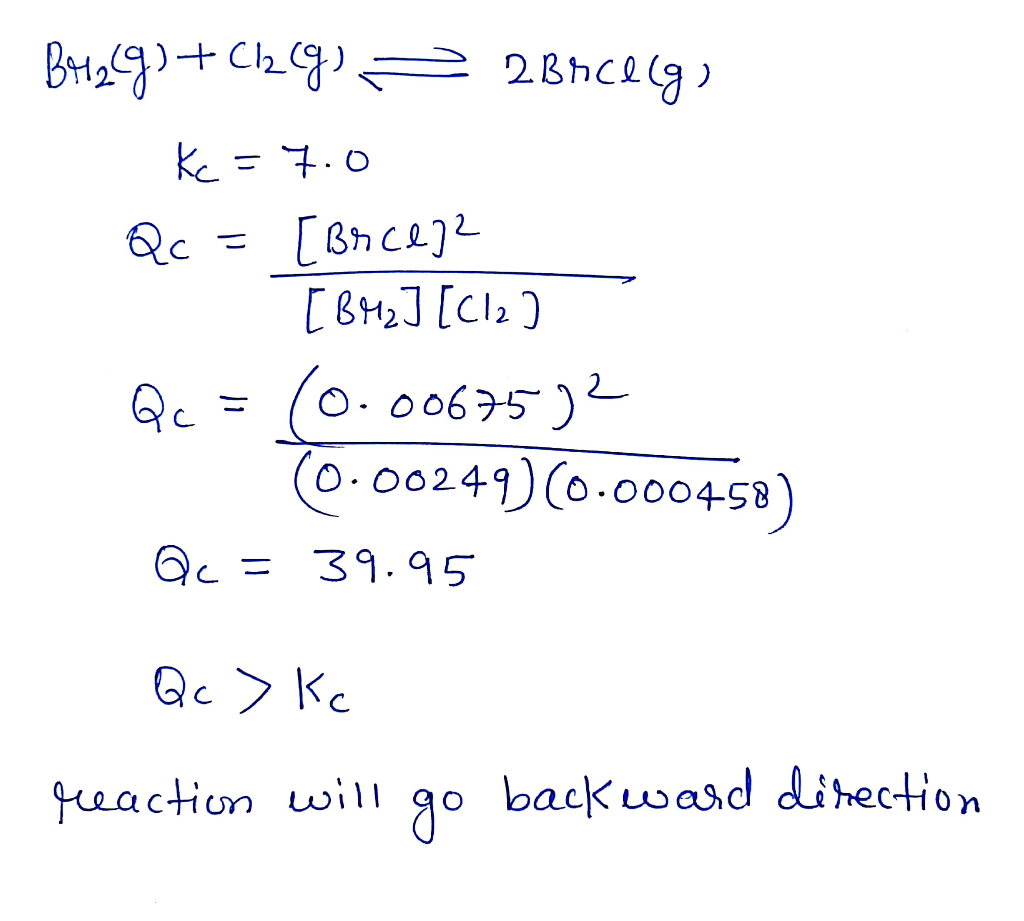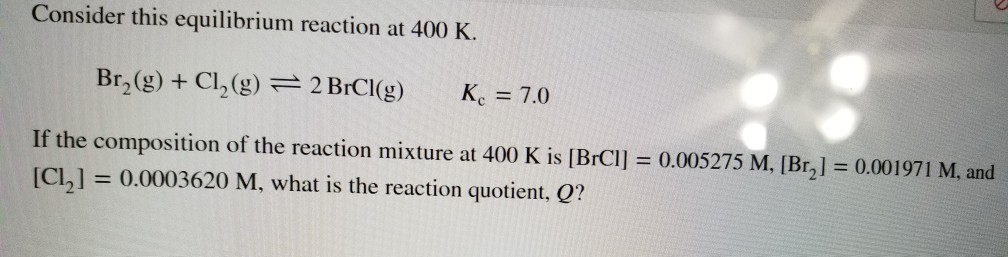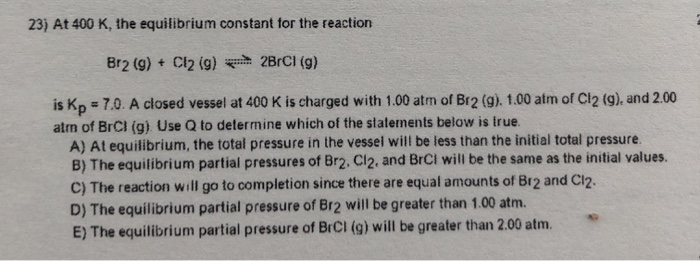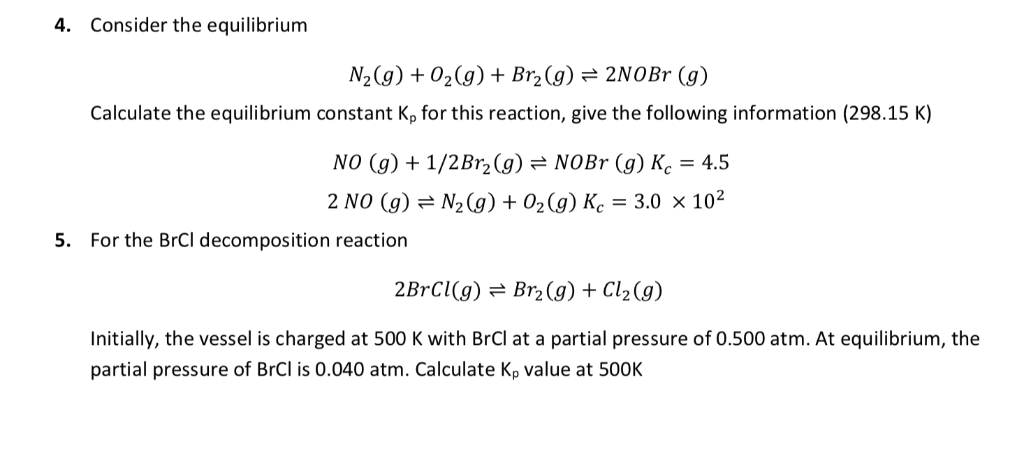Question

# Consider this equilibrium reaction at 400 K. Br2(g)+Cl2(g)↽−−⇀2BrCl(g)?c=7.0 If the composition of the reaction mixture at...

Consider this equilibrium reaction at 400 K.

Br2(g)+Cl2(g)↽−−⇀2BrCl(g)?c=7.0

If the composition of the reaction mixture at 400 K is [BrCl]=0.00675, [Br2]=0.00249, and [Cl2]=0.000458, what is the reaction quotient, ?Q?

?=

How is the reaction quotient related to the equilibrium constant, Kc, for this reaction?#### Earn Coins

Coins can be redeemed for fabulous gifts.

Similar Homework Help Questions
• ### Consider this equilibrium reaction at 400 K. Br2(g) + Cl2(g) = 2 BrCl(g) K. = 7.0...Consider this equilibrium reaction at 400 K. Br2(g) + Cl2(g) = 2 BrCl(g) K. = 7.0 If the composition of the reaction mixture at 400 K is [BrCl] = 0.005275 M, [Br] = 0.001971 M, and [CL] = 0.0003620 M, what is the reaction quotient, Q?

• ### At 400 K, the equilibrium constant for the reaction Br2 (g) + Cl2 (g) <=> 2BrCl...

At 400 K, the equilibrium constant for the reaction Br2 (g) + Cl2 (g) <=> 2BrCl (g) is Kp = 7.0. A closed vessel at 400 K is charged with 1.00 atm of Br2 (g), 1.00 atm of Cl2 (g), and 2.00 atm of BrCl (g). What is the equilibrium pressure of Br2? A. 0.86 atm B. The equilibrium partial pressures of Br2 will be the same as the initial value. C. The equilibrium partial pressure of Br2 will be...

• ### For the equilibrium Br2(g)+Cl2(g)⇌2BrCl(g) at 400 K, Kc = 7.0. Part A If 0.25 mol of...

For the equilibrium Br2(g)+Cl2(g)⇌2BrCl(g) at 400 K, Kc = 7.0. Part A If 0.25 mol of Br2 and 0.55 mol of Cl2 are introduced into a 3.0-L container at 400 K, what will be the equilibrium concentration of Br2? Express your answer to two significant figures and include the appropriate units. Part B If 0.25 mol of Br2 and 0.55 mol of Cl2 are introduced into a 3.0-L container at 400 K, what will be the equilibrium concentration of Cl2?...

• ### For the equilibrium Br2(g)+Cl2(g)⇌2BrCl(g) at 400 K, Kc = 7.0. Part A If 0.25 mol of...

For the equilibrium Br2(g)+Cl2(g)⇌2BrCl(g) at 400 K, Kc = 7.0. Part A If 0.25 mol of Br2 and 0.55 mol of Cl2 are introduced into a 3.0-L container at 400 K, what will be the equilibrium concentration of Br2? Express your answer to two significant figures and include the appropriate units. Part B If 0.25 mol of Br2 and 0.55 mol of Cl2 are introduced into a 3.0-L container at 400 K, what will be the equilibrium concentration of Cl2?...

• ### 23) At 400 K, the equilibrium constant for the reaction Br2(g) + Cl2 (9) 2BrCl (9)...23) At 400 K, the equilibrium constant for the reaction Br2(g) + Cl2 (9) 2BrCl (9) is Kp = 70. A closed vessel at 400 K is charged with 1.00 atm of Br2 (g) 1.00 atm of Cl2 (g), and 2.00 alm of BrCl (g). Use Q to determine which of the statements below is true. A) At equilibrium, the total pressure in the vessel will be less than the initial total pressure. B) The equilibrium partial pressures of Br2....

• ### At 400K, the equilibrium constant for the reaction Br2(g) + Cl2(g) ⇌ 2BrCl(g) is KP =...

At 400K, the equilibrium constant for the reaction Br2(g) + Cl2(g) ⇌ 2BrCl(g) is KP = 7.0. A closed vessel at 400K is charged with 1.00 atm of Br2(g), 1.00 atm of Cl2(g), and 2.00 atm of BrCl(g). Use Q to determine which of the statements below is true. A. The equilibrium partial pressure of BrCl(g) will be less than 2.00 atm B. The reaction will go to completion since there are equal amounts of Br2 and Cl2 C. At...

• ### For the reaction given below, the value of the equilibrium constant at 400K is 7.0 Br2(g)...

For the reaction given below, the value of the equilibrium constant at 400K is 7.0 Br2(g) + Cl2(g) ßà 2BrCl(g) At equilibrium the concentration of Br2 and Cl2 are each 1 mol/L. What is the equilibrium concentration of BrCl? a.       49 mol/L b.       1.0 mol/L c.       7.0 mol/L d.       2.6 mol/L

• ### If 0.25 mol of Br2 and 0.55mol of Cl2 are introduced into a 3.0-L container at...

If 0.25 mol of Br2 and 0.55mol of Cl2 are introduced into a 3.0-L container at 400 K, what will be the equilibrium concentration of BrCl ?? Br2(g)+Cl2(g) 2BrCl at 400 K, Kc= 7.0

• ### In an analysis of the following reaction at a certain temperature, Br2(g) + Cl2(g) ⇌ 2BrCl(g)...

In an analysis of the following reaction at a certain temperature, Br2(g) + Cl2(g) ⇌ 2BrCl(g) the equilibrium concentrations were found to be [Br2] = 3.5 × 10−3 M, [Cl2] = 1.6 × 10−2 M, and [BrCl] = 1.9 × 10−2 M. Write the equilibrium expression, and calculate the equilibrium constant for this reaction at this temperature.

• ### Consider the equilibrium 4. N2(g) 02(g) Br2(g) 2NOBr (g) Calculate the equilibrium constant Kp for this reaction, give...Consider the equilibrium 4. N2(g) 02(g) Br2(g) 2NOBr (g) Calculate the equilibrium constant Kp for this reaction, give the following information (298.15 K) NO (g) +1/2Br2(g) NOBr(g) Ke 4.5 2 NO (g)N2(g) 02(g) Ke 3.0 x 102 5. For the BrCl decomposition reaction 2BrCl(g) Br2(g Cl2(g) Initially, the vessel is charged at 500 K with BrCl at a partial pressure of 0.500 atm. At equilibrium, the partial pressure of BrC is 0.040 atm. Calculate Kp value at 500K Consider the...